### Probability Class 10th Mathematics CBSE Solution

##### Question 1.Two customers Shyam and Ekta are visiting a particular shop in the same week (Tuesday to Saturday). Each is equally likely to visit the shop on any day as on another day. What is the probability that both will visit the shop on(i) The same day?(ii) Consecutive days?(iii) Different days?Answer:There are a total of 5 days. Shyam can go to the shop in 5 ways and Ekta can go to the shop in 5 ways.Therefore, total number of outcomes = 5 × 5 = 25(i) They can reach on the same day in 5 ways.i.e., (t, t), (w, w), (th, th), (f, f), (s, s)P (both will reach on same day) =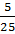=(ii) They can reach on consecutive days in these 8 ways - (t, w), (w, th), (th, f), (f, s), (w, t), (th, w), (f, th), (s, f)Therefore, P (both will reach on consecutive days) =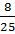(iii) P (both will reach on same day)=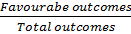=P (both will reach on different days) = 1 -=Question 2.A die is numbered in such a way that its faces show the numbers 1, 2, 2, 3, 3, 6. It is thrown two times and the total score in two throws is noted. Complete the following table which gives a few values of the total score on the two throws: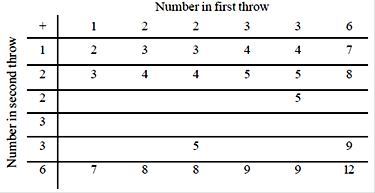What is the probability that the total score is(i) Even?(ii) 6?(iii) At least 6?Answer:Total number of possible outcomes when two dice are thrown = 6 × 6 = 36(i) Total times when the sum is even = 18P (getting an even number) ==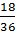=(ii) Total times when the sum is 6 = 4P (getting sum as 6) ==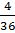=(iii) Total times when the sum is at least 6 (i.e., greater than 5) = 15P (getting sum at least 6) ==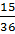=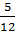Question 3.A bag contains 5 red balls and some blue balls. If the probability of drawing a blue ball is double that of a red ball, determine the number of blue balls in the bagAnswer:Let the number of blue balls be x.Number of red balls = 5Total number of balls = x + 5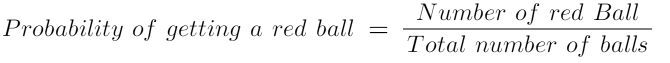P (getting a red ball) =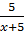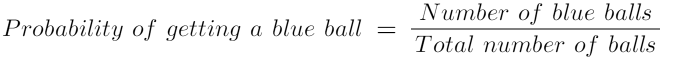P (getting a blue ball) =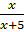It is given that,Probability of getting a blue ball is two times the probability of getting a red ball. Therefore,Hence, number of blue balls = 10Question 4.A box contains 12 balls out of which x are black. If one ball is drawn at random from the box, what is the probability that it will be a black ball?If 6 more black balls are put in the box, the probability of drawing a black ball is now double of what it was before. Find xAnswer:Total number of balls = 12Total number of black balls = xP (getting a black ball) =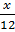If 6 more black balls are put in the box, thenTotal number of balls = 12 + 6 = 18Total number of black balls = x + 6P (getting a black ball now) =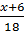According to the given condition,2 () =3x = x + 62x = 6x = 3Question 5.A jar contains 24 marbles, some are green and others are blue. If a marble is drawn at random from the jar, the probability that it is green is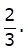Find the number of blue balls in the jarAnswer:Total number of marbles = 24Let the total number of green marbles be xThen, total number of blue marbles = 24 - xP (getting a green marble) =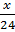According to the condition given in the question,=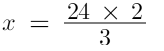x = 16Therefore, total number of green marbles in the jar = 16Hence, total number of blue marbles = 24 - x= 24 - 16= 8

PDF FILE TO YOUR EMAIL IMMEDIATELY PURCHASE NOTES & PAPER SOLUTION. @ Rs. 50/- each (GST extra)

HINDI ENTIRE PAPER SOLUTION

MARATHI PAPER SOLUTION

SSC MATHS I PAPER SOLUTION

SSC MATHS II PAPER SOLUTION

SSC SCIENCE I PAPER SOLUTION

SSC SCIENCE II PAPER SOLUTION

SSC ENGLISH PAPER SOLUTION

SSC & HSC ENGLISH WRITING SKILL

HSC ACCOUNTS NOTES

HSC OCM NOTES

HSC ECONOMICS NOTES

HSC SECRETARIAL PRACTICE NOTES

# 2019 Board Paper Solution

HSC ENGLISH SET A 2019 21st February, 2019

HSC ENGLISH SET B 2019 21st February, 2019

HSC ENGLISH SET C 2019 21st February, 2019

HSC ENGLISH SET D 2019 21st February, 2019

SECRETARIAL PRACTICE (S.P) 2019 25th February, 2019

HSC XII PHYSICS 2019 25th February, 2019

CHEMISTRY XII HSC SOLUTION 27th, February, 2019

OCM PAPER SOLUTION 2019 27th, February, 2019

HSC MATHS PAPER SOLUTION COMMERCE, 2nd March, 2019

HSC MATHS PAPER SOLUTION SCIENCE 2nd, March, 2019

SSC ENGLISH STD 10 5TH MARCH, 2019.

HSC XII ACCOUNTS 2019 6th March, 2019

HSC XII BIOLOGY 2019 6TH March, 2019

HSC XII ECONOMICS 9Th March 2019

SSC Maths I March 2019 Solution 10th Standard11th, March, 2019

SSC MATHS II MARCH 2019 SOLUTION 10TH STD.13th March, 2019

SSC SCIENCE I MARCH 2019 SOLUTION 10TH STD. 15th March, 2019.

SSC SCIENCE II MARCH 2019 SOLUTION 10TH STD. 18th March, 2019.

SSC SOCIAL SCIENCE I MARCH 2019 SOLUTION20th March, 2019

SSC SOCIAL SCIENCE II MARCH 2019 SOLUTION, 22nd March, 2019

XII CBSE - BOARD - MARCH - 2019 ENGLISH - QP + SOLUTIONS, 2nd March, 2019

# HSCMaharashtraBoardPapers2020

(Std 12th English Medium)

HSC ECONOMICS MARCH 2020

HSC OCM MARCH 2020

HSC ACCOUNTS MARCH 2020

HSC S.P. MARCH 2020

HSC ENGLISH MARCH 2020

HSC HINDI MARCH 2020

HSC MARATHI MARCH 2020

HSC MATHS MARCH 2020

# SSCMaharashtraBoardPapers2020

(Std 10th English Medium)

English MARCH 2020

HindI MARCH 2020

Hindi (Composite) MARCH 2020

Marathi MARCH 2020

Mathematics (Paper 1) MARCH 2020

Mathematics (Paper 2) MARCH 2020

Sanskrit MARCH 2020

Sanskrit (Composite) MARCH 2020

Science (Paper 1) MARCH 2020

Science (Paper 2)

Geography Model Set 1 2020-2021

MUST REMEMBER THINGS on the day of Exam

Are you prepared? for English Grammar in Board Exam.

Paper Presentation In Board Exam

How to Score Good Marks in SSC Board Exams

Tips To Score More Than 90% Marks In 12th Board Exam

How to write English exams?

How to prepare for board exam when less time is left

How to memorise what you learn for board exam

No. 1 Simple Hack, you can try out, in preparing for Board Exam

How to Study for CBSE Class 10 Board Exams Subject Wise Tips?

JEE Main 2020 Registration Process – Exam Pattern & Important Dates

NEET UG 2020 Registration Process Exam Pattern & Important Dates

How can One Prepare for two Competitive Exams at the same time?

8 Proven Tips to Handle Anxiety before Exams!